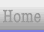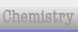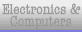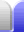# Mathematics Glossary

Argand diagram – a way of representing complex numbers, where the real component is on the horizontal axis and the imaginary component is on the vertical axis.

Bisect – to split evenly in two. The resulting set of points is a line called the bisector that is equidistant from whatever was being bisected. If a line is bisected the result is a line perpendicular to the bisected line and passes through the line's midpoint. If an angle is bisected the angle is split in two. The bisector is equidistant from the two original lines that make the angle.

Bisector – a line equidistant from two other lines or points.

Cartesian plane – a way of representing points in two dimensions so that the x value is on the horizontal axis and the y value is on the vertical axis.

Centroid – the barycentre, or centre of mass of a triangle, found by taking a line from each vertex of the triangle to the midpoint of the opposing side; see Triangle Centres. Barycentre is also used in astronomy.

Circumcentre – the point in a triangle that is the centre of the circumcircle; see Triangle Centres.

Circumcircle – the circle drawn outside a triangle, so that the circle touches all three points of the triangle; see Triangle Centres.

Counting numbers – see natural numbers below.

Denominator – the number of the bottom of a fraction. (I remember it because denominator starts with d for down.)

Factor – numbers which are multiplied together; two or more factors multiply together to make a product.

Improper fraction – a fraction with the numerator larger than the denominator. For example, 8/3.

Incentre – the point in a triangle that is the centre of the incircle; see Triangle Centres.

Incircle – the circle drawn inside a triangle, so that the circle touches all three sides of the triangle; see Triangle Centres.

Integers – all natural numbers, zero, and negative versions of all of them as well: ..., -5, -4, -3, -2, -1, 0, 1, 2, 3, 4, 5, ...

Irrational numbers – numbers which cannot be made as a fraction; see Number Sets.

Natural numbers – the numbers from 1 upward: 1, 2, 3, 4, 5, ...; see Number Sets.

Numerator – the number on the top of a fraction.

Orthocentre – the point found by taking the perpendicular line through each side of the triangle which passes through the opposing vertex of the triangle; see Triangle Centres.

Perimeter – the outer edge of a shape, or the distance around the outside of the shape.

Prime number – a number greater than 1 which only has the factors 1 and itself; see Prime Numbers.

Product – the result of multiplying numbers together; two or more factors multiply together to make a product.

Quadrilateral – a four sided polygon.

Rational number – a number which can be made as a fraction; see Number Sets.

Regular – a regular polygon has all its sides the same length and all its angles the same size.

Sieve of Eratosthenes – a method of finding prime numbers; see Prime Numbers.

Sum – the result of adding two or more numbers together, or sometimes the process of doing the adding.

Tangent – a line that touches a curve (such as a circle) at one point only. A tangent to a circle is perdendicular to the radius at the point where the tangent touches the circle.

Triangle – a three sided polygon; see Triangles.

Vertex – a point or corner of a polygon.

Whole number – the numbers from 0 upward: 0, 1, 2, 3, 4, ...; see Number Sets.Home Astronomy Chemistry Electronics Mathematics Physics Field Trips Turn on javascript for email link